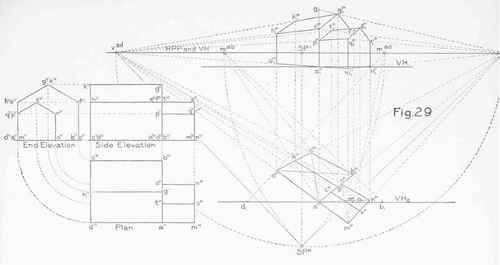112. Perspective is essentially a science of straight lines. If curved lines occur in a problem, the simplest way to find their perspective is to refer the curves to straight lines.

If the curve is of simple, regular form, such as a circle or an ellipse, it may be enclosed in a rectangle. The perspective of the enclosing rectangle may then be found. A curve inscribed within this perspective rectangle will be the perspective of the given curve.

Fig. 30 shows a circle inscribed in a square. The points of intersection of the diameters with the sides of the square give the four points of tangency between the square and circle. The sides of the square give the directions of the circle at these points. Additional points on the circle may be established by drawing the diagonals of the square, and through the points mH, kh nh, and hH drawing construction lines parallel to the sides of the square, as indicated in the figure. Fig. 31 shows the square, which is supposed to lie in a horizontal plane, in parallel perspective. One side of the square (apdp) lies in the picture plane, and will show in its true size. The vanishing point for the sides perpendicular to the picture plane will coincide with SPV (§ 52, note). The measure point for these sides has been found at mab, in accordance with principles already explained, ap b1 is laid off on VH1 to the right of the point ap, equal to the true length of the side of the square. A measure line through b1, vanishing at mab, will determine the position of the point bp. bp cp ill be parallel to apdp (§ 54, note).

Fig.30.Fig.3l.The diagonals of the square may be drawn. Their intersection will determine the perspective center of the square. The diameters will pass through this perspective center, one vanishing at SPV, and the other being parallel to apdp (§ 54, note).

The divisions dpep and apfp will show in their true size. Lines through ep and f p, vanishing at SPV, will intersect the diagonals of the square, giving four points on the perspective of the circle. Four other points on the perspective of the circle will be determined by the intersections of the diameter with the sides of the square. The perspective of the curve can be drawn as indicated.

113. If the curve is of very irregular form, such as that shown in Fig. 32, it can be enclosed in a rectangle, and the rectangle divided by lines, drawn parallel to its sides, into smaller rectangles, as indicated in the figure.

The perspective of the rectangle with its dividing lines may then be found, and the perspective of the curve drawn in free hand. This is shown in Fig. 33. If very great accuracy is required, the perspectives of the exact points where the curve crosses the dividing lines of the rectangle may be found.

Fig.32.